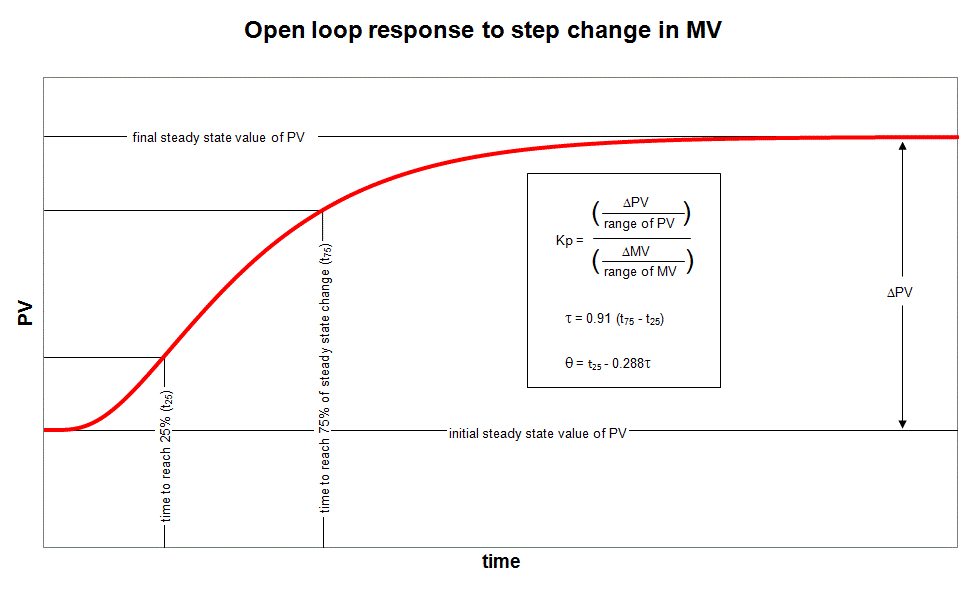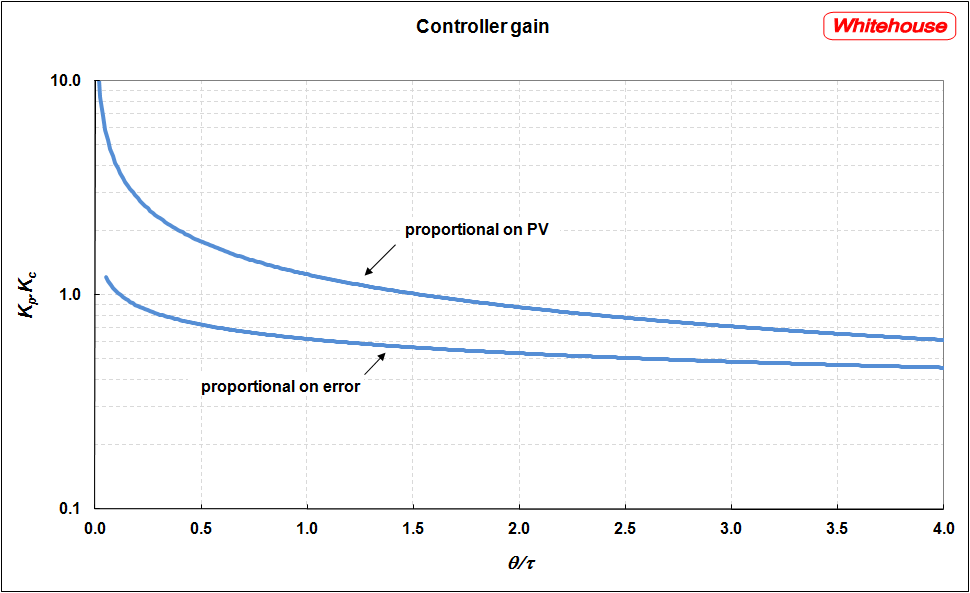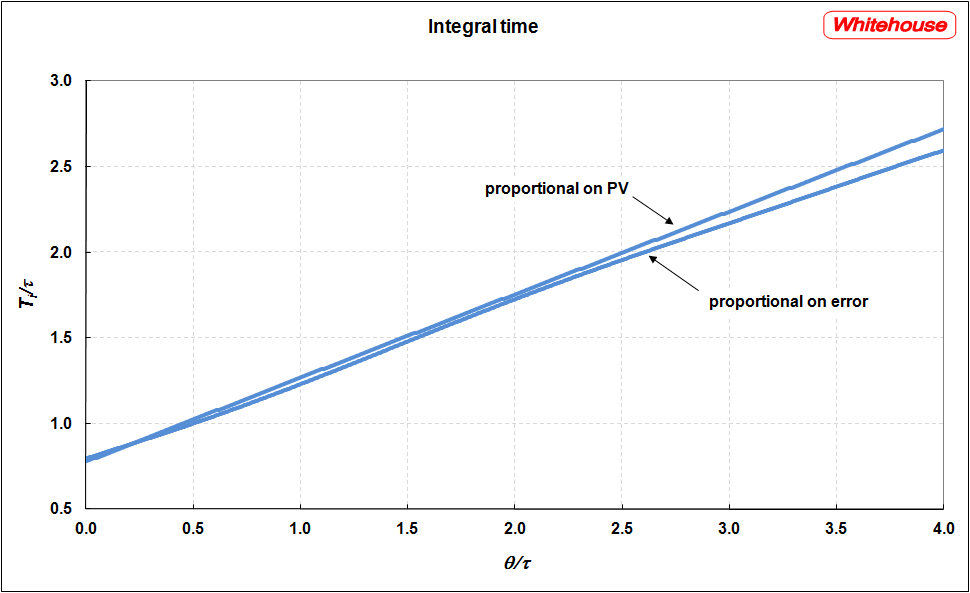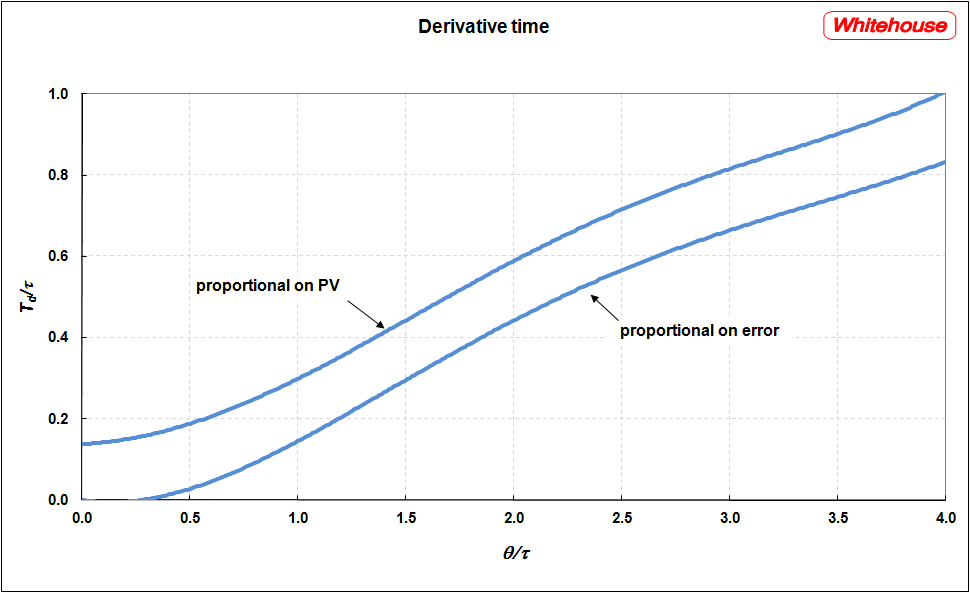The tuning package developed by Whitehouse will tune almost any type of controller using a variety of tuning methods. It comprises two parts. Model identification imports process data from a variety of sources (usually an Excel spreadsheet). It can model both self-regulating and integrating processes, generating a first order plus deadtime (FOPDT) model. The Tuner uses this model to determine PID tuning constants. This part can be downloaded for unrestricted use.

After installation you may get an error that MSVBVM50.DLL is missing. This can be resolved by downloading it from

In addition to the more common tuning methods it offers a well-proven technique developed by Whitehouse. This comprises a large collection of tuning charts, an example of which is presented below. This set is applicable only under the following conditions:

• the full PID algorithm is used, not PI or P only
• the standard non-interactive control algorithm is used
• the derivative action is based on PV (process variable), not on error
• the controller scan interval is less than 2% of the process lag
• tuning is for SP rather than load changes
• tuning is required to minimise ITAE (integral over time of absolute error) subject to an MV overshoot limit of 15%

To derive the tuning constants using the charts, the process dynamics must first be known. Whitehouse's package will derive these from almost any disturbance. However they can be approximated by performing an open loop step test. The result of this test is best analysed as follows:There is a tuning chart for each of the tuning constants - controller gain (Kc), integral time (Ti) and derivative time (Td). Tuning for both the proportional on PV and proportional on error algorithms is given. If available, Whitehouse recommends the use of proportional on PV.

Take care with units. If Ti and Td are in minutes then t25 and t75 should be determined in minutes. If proportional band is required, this is given by 100/Kc.